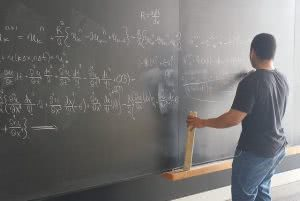Why You Should Memorize SAT Exam Math FormulasFlickr user Bryan Alexander

For many students, the math section is the most dreaded part of the SAT exam. The fact that the math formulas are provided on the test paper offers some degree of relief and hopefully one less thing to worry about during test prep.

Before you rejoice at the news. You should know that it’s strongly advised to memorize SAT math formulas even though they are provided at the time of taking the test. Here’s why…

Time is of the Essence on the SAT Exam

The SAT is a timed test. Most students find themselves racing against the clock to answer all the test questions. When taking the math section if you spend precious seconds turning over your test paper to look up every formula, you run the risk of running out of time. It would be a shame to lose marks only because you ran out of time and submitted an incomplete test paper.

Taking the time to memorize some of the formulas during your SAT prep can save you precious time during the test. This will give you more time to answer all questions and even check over your paper, increasing your chances of getting a higher score.

How to Memorize Math Formulas

First thing’s first: you’ll need to know what sort of math questions are asked on the SAT. There are three main categories—algebra, problem solving & data analysis, and advanced math. We go into depth about the SAT math breakdown in this article.

ACT takers are no strangers to memorizing math formulas, as that test doesn’t provide formulas. SAT takers can take advantage of their ACT studying methods when it comes to memorizing math equations. The best way to understand them is to practice them. There are plenty of math test prep resources online and through books or school math reviews.

Keep in mind that the SAT has a calculator and non-calculator section, so don’t fully rely on your machine doing all the work for you. It’s important to know how to work through each formula for the SAT exam.

Check out how your SAT score affects your acceptance odds with College Raptor!